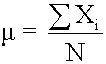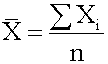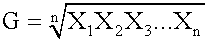# on 30-Mar-2016 (Wed)

#### Flashcard 1331948031244

Question
The Old Generation is used to store l
[default - edit me]

status measured difficulty not learned 37% [default] 0
Java Garbage Collection Basics
d Event - All minor garbage collections are "Stop the World" events. This means that all application threads are stopped until the operation completes. Minor garbage collections are always Stop the World events. <span>The Old Generation is used to store long surviving objects. Typically, a threshold is set for young generation object and when that age is met, the object gets moved to the old generation. Eventually the old generation need

#### Annotation 1331958779148

 Subject 4. Measures of Center Tendency #has-images #quantitative-methods-basic-concepts #statistics Measures of central tendency specify where data are centered. They attempt to use a typical value to represent all the observations in the data set. Population Mean The population mean is the average for a finite population. It is unique; a given population has only one mean.where: N = the number of observations in the entire populationXi = the ith observationΣXi = add up Xi, where i is from 0 to N Sample Mean The sample mean is the average for a sample. It is a statistic and is used to estimate the population mean.where n = the number of observations in the sample Arithmetic Mean The arithmetic mean is what is commonly called the average. The population mean and sample mean are both examples of the arithmetic mean. If the data set encompasses an entire population, the arithmetic mean is called a population mean.If the data set includes a sample of values taken from a population, the arithmetic mean is called a sample mean. This is the most widely used measure of central tendency. When the word "mean" is used without a modifier, it can be assumed to refer to the arithmetic mean. The mean is the sum of all scores divided by the number of scores. It is used to measure the prospective (expected future) performance (return) of an investment over a number of periods. All interval and ratio data sets (e.g., incomes, ages, rates of return) have an arithmetic mean.All data values are considered and included in the arithmetic mean computation.A data set has only one arithmetic mean. This indicates that the mean is unique.The arithmetic mean is the only measure of central tendency where the sum of the deviations of each value from the mean is always zero. Deviation from the arithmetic mean is the distance between the mean and an observation in the data set. The arithmetic mean has the following disadvantages: The mean can be affected by extremes, that is, unusually large or small values.The mean cannot be determined for an open-ended data set (i.e., n is unknown). Geometric MeanThe geometric mean has three important properties: It exists only if all the observations are greater than or equal to zero. In other words, it cannot be determined if any value of the data set is zero or negative.If values in the data set are all equal, both the arithmetic and geometric means will be equal to that value.It is always less than the arithmetic mean if values in the data set are not equal. It is typically used when calculating returns over multiple periods. It is a better measure of the compound growth rate of an investment. When returns are variable by period, the geometric mean will always be less than the arithmetic mean. The more dispersed the rates of returns, the greater the difference between the two. This measurement is not as highly influenced by extreme values as the arithmetic mean. Weighted Mean The weighted mean is computed ...